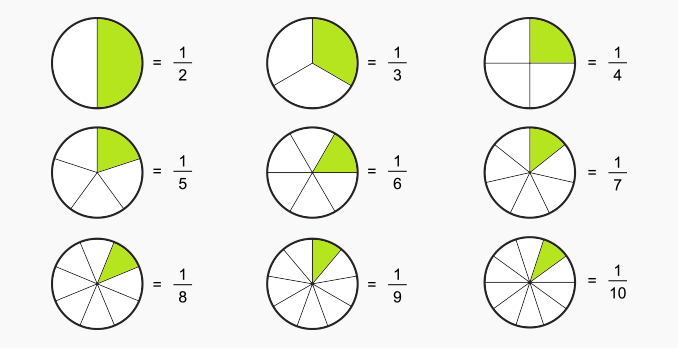Subtract Fractions With Unlike Denominators - Definition with Examples

The Complete K-5 Math Learning Program Built for Your Child

• 30 Million Kids

Loved by kids and parent worldwide

• 50,000 Schools

Trusted by teachers across schools

• Comprehensive Curriculum

Aligned to Common Core

Subtract Fractions with Unlike Denominators

Fractions with unlike denominators are the fractions having different denominators.For instance, fractions 1 4 and 1 3 are unlike denominators.

Another example is if Tom has 3 4 of a pizza and he eats 1 8 We need to take away 1 8 from 3 4 to work out how much pizza did he eat.

Similarly, if Macy had a half of an apple and she ate one quarter of it in the morning. To work out the remaining portion of the apple left, we need to take away from  1 4 from 1 2 .

Steps to subtract fractions with unlike denominators:

(i) Identify the unlike fractions we need to subtract.

(ii) Convert both the fractions into their equivalent fractions such that they have like denominators.

(iii) Replace both the fractions in Step (i) by their equivalent fractions obtained in Step(ii).

(iv) As we have fractions with like denominators, we can subtract the numerators directly to obtain the answer and the denominator remains the  same.

(v) Simplify the fraction obtained in Step (iv) if possible.

Case-1: When one of the denominators is a multiple of the other.

Example 1: Work out: 1 2 - 2 8 .

Solution:

 Step Number Observation Working Out Step (i) Unlike Denominators 1 2 - 2 8 Step (ii) The common denominator for the above fractions is 8. Working out the equivalent fractions. 1 × 4 2 × 4 = 4 8 ; 2 8 = 2 8 Step (iii) Replacing the fractions in (i) by equivalent fractions from (ii) 4 8 - 2 8 Step (iv) We have like denominators now. So, we directly take away the numerators. 4 8 - 2 8 = 2 8 Step (v) Simplifying the answer from (iv) 2 ÷ 2 8 ÷ 2 = 1 4

Hence, 1 2 - 2 8 = 1 4

Case-2: When both the denominators are different.

Example 2: Jack has of 4 5 of a papaya. If he gives 1 3 of it to Lucy, what fraction of papaya is left with Jack?

Solution:

 Step Number Observation Working Out Step (i) Unlike Denominators 4 5 - 1 3 Step (ii) The common denominator for the above fractions is 15. Working out the equivalent fractions. 4 × 3 5 × 3 = 12 15 ; 1 × 5 3 × 5  =  5 15 Step (iii) Replacing the fractions in (i) by equivalent fractions from (ii) 12 15 - 5 15 Step (iv) We have like denominators now. So, we directly take away the numerators. 12 15 - 5 15 = 7 15 Step (v) The answer is already in its simplest form. 2 ÷ 2 8 ÷ 2 = 1 4

Hence, Jack has 1 4  of the papaya left.

Example 3: Allie and Joseph are competing in a bicycle race. If Allie has covered 3 4 of the total distance and Joseph has covered 1 5 of the total distance. What is the difference in the distance covered by both of them?

Solution:

 Step Number Observation Working Out Step (i) Unlike Denominators 3 4 - 1 5 Step (ii) The common denominator for the above fractions is 20. Working out the equivalent fractions. 3 × 5 4 × 5 = 15 20 ; 1 × 4 5 × 4 = 4 20 Step (iii) Replacing the fractions in (i) by equivalent fractions from (ii) 15 20 - 4 20 Step (iv) We have like denominators now. So, we directly take away the numerators. 15 20 - 4 20 = 11 20 Step (v) The answer is already in its simplest form. 11 20

Hence, the difference between the distance covered by Allie and Joseph is 11 20  of the total distance.

 Fun Facts Every whole number can be written as a fraction.           For instance:  2 can be written as  2 1 or 3 as 3 1  and so on.

Won Numerous Awards & Honors